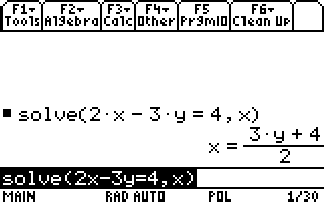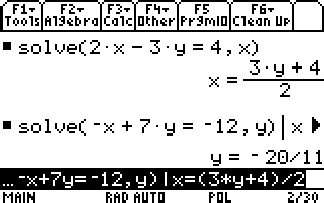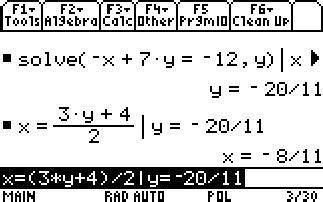# Knowledge Base

## Solution 12159: Solving Systems of Equations Using Symbolic Manipulation on the TI-89 Family, TI-92 Family and Voyage™ 200 Graphing Calculators.

### How can I solve a system of equations on the TI-89 family, TI-92 family, or Voyage 200 using symbolic manipulation?

The example below shows how to solve a system of equations using the symbolic manipulation capabilities of the TI-89 family, TI-92 family, and Voyage 200.

Example: Solve the system of equations 2x-3y=4 and -x+7y=-12.

Solve the first equation so that x is expressed in terms of y. Substitute x into the second equation, and solve for the value of y. Then, substitute the y value back into the first equation to solve for the value of x.

From the Home screen:

• Press [F2].
• Select 1:Solve(
• Input the equation as follows: solve(2x-3y=4,x) and press [ENTER].

The results x=(3y+4)/2 will appear on the Home screen.• Press [F2].
• Select 1:Solve(
• Input the equation as follows: solve(-x+7y=-12,y)
• Press [|] (the "with" operator) to substitute the expression for x  This gives the value for y.
• Move the top arrow key to highlight calculated expression for x.

x=(3y+4)/2

To copy the equation press [F1] and select 5:Copy.  Paste the calculated expression for x on the entry line by pressing [F1] and 6:Paste.  Your command line should read:

solve(-x+7y=-12,y)|x=(3y+4)/2

• Press [ENTER] and the screen will display y=-20/11.• Press the up arrow key to highlight the equation for x.

x=(3y+4)/2.

• Now copy and paste this equation into the entry line.
• Press [|]
• Now move the top arrow key to highlight calculated expression for y.

y=-20/11

• Copy and paste this equation after the "with" command.
• Press [ENTER] and the screen will display x=-8/11.The solution is: x=-8/11 and y=-20/11.

Please see the TI-89 family, TI-92 family, and Voyage 200 guidebooks for additional information.